# Plot Multiple lines in Matplotlib

In this article, we will learn how to plot multiple lines using matplotlib in Python. Let’s discuss some concepts:

• Matplotlib: Matplotlib is an amazing visualization library in Python for 2D plots of arrays. Matplotlib is a multi-platform data visualization library built on NumPy arrays and designed to work with the broader SciPy stack. It was introduced by John Hunter in the year 2002.
• Line plot: Line plots can be created in Python with Matplotlib’s pyplot library. To build a line plot, first import Matplotlib. It is a standard convention to import Matplotlib’s pyplot library as plt. The plt alias will be familiar to other Python programmers.

Here we will discuss some examples to draw a line or multiple lines with different features. To do such work we must follow the steps given below:

• Import libraries.
• Create Data.
• Plot the lines over data.

## Plotting a single Horizontal Line

In this example, we will learn how to draw a horizontal line with the help of matplotlib. Here we will use two lists as data for two dimensions (x and y) and at last plot the line. For making a horizontal line we have to change the value of the x-axis continuously by taking the y-axis as constant.

## Python3

 `# importing package ` `import` `matplotlib.pyplot as plt ` ` `  `# create data ` `x ``=` `[``10``,``20``,``30``,``40``,``50``] ` `y ``=` `[``30``,``30``,``30``,``30``,``30``] ` ` `  `# plot line ` `plt.plot(x, y) ` `plt.show()`

Output: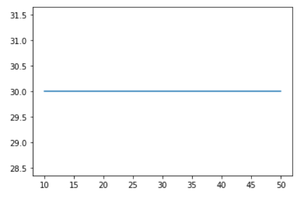## Plotting a single Vertical Line

In this example, we will learn how to draw a vertical line with the help of matplotlib. Here we will use two lists as data with two dimensions (x and y) and at last plot the line. For making a vertical line we have to change the value of the y-axis continuously by taking the x-axis as constant. So we change the axes to get a vertical line.

## Python3

 `# importing package ` `import` `matplotlib.pyplot as plt ` ` `  `# create data ` `x ``=` `[``10``,``20``,``30``,``40``,``50``] ` `y ``=` `[``30``,``30``,``30``,``30``,``30``] ` ` `  `# plot line ` `plt.plot(y,x) ` `plt.show()`

Output: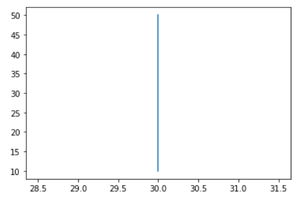## Plotting a Horizontal and a Vertical Line

In this example, we will learn how to draw a horizontal line and a vertical line both in one graph with the help of matplotlib. Here we will use two list as data with two dimensions (x and y) and at last plot the line with respect to the dimensions. So, in this example we merge the above both graphs to make both lines together in a graph.

## Python3

 `# importing package ` `import` `matplotlib.pyplot as plt ` ` `  `# create data ` `x ``=` `[``10``,``20``,``30``,``40``,``50``] ` `y ``=` `[``30``,``30``,``30``,``30``,``30``] ` ` `  `# plot lines ` `plt.plot(x, y, label ``=` `"line 1"``) ` `plt.plot(y, x, label ``=` `"line 2"``) ` `plt.legend() ` `plt.show()`

Output: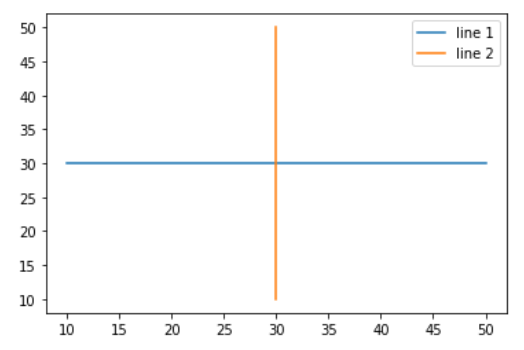## Plotting Multiple Lines

In this example, we will learn how to draw multiple lines with the help of matplotlib. Here we will use two lists as data with two dimensions (x and y) and at last plot the lines as different dimensions and functions over the same data.

To draw multiple lines we will use different functions which are as follows:

• y = x
• x = y
• y = sin(x)
• y = cos(x)

## Python3

 `# importing package ` `import` `matplotlib.pyplot as plt ` `import` `numpy as np ` ` `  `# create data ` `x ``=` `[``1``,``2``,``3``,``4``,``5``] ` `y ``=` `[``3``,``3``,``3``,``3``,``3``] ` ` `  `# plot lines ` `plt.plot(x, y, label ``=` `"line 1"``) ` `plt.plot(y, x, label ``=` `"line 2"``) ` `plt.plot(x, np.sin(x), label ``=` `"curve 1"``) ` `plt.plot(x, np.cos(x), label ``=` `"curve 2"``) ` `plt.legend() ` `plt.show()`

Output: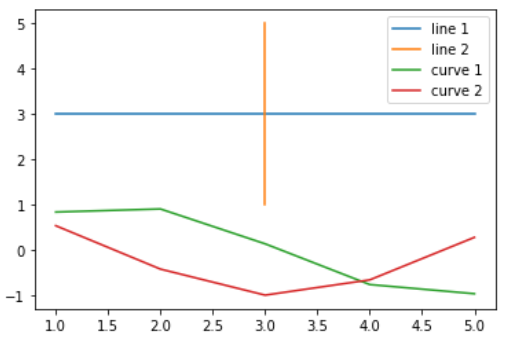## Plotting Multiple Lines with different Line styles

This example is similar to the above example and the enhancement is the different line styles. This can help in the modification of better visualization.

Here we will use different line styles which are as follows:

• –        : dashed
•      : double dashed
• -.       : dashed-dotted
•       : dotted

## Python3

 `# importing package ` `import` `matplotlib.pyplot as plt ` `import` `numpy as np ` ` `  `# create data ` `x ``=` `[``1``,``2``,``3``,``4``,``5``] ` `y ``=` `[``3``,``3``,``3``,``3``,``3``] ` ` `  `# plot lines ` `plt.plot(x, y, label ``=` `"line 1"``, linestyle``=``"-"``) ` `plt.plot(y, x, label ``=` `"line 2"``, linestyle``=``"--"``) ` `plt.plot(x, np.sin(x), label ``=` `"curve 1"``, linestyle``=``"-."``) ` `plt.plot(x, np.cos(x), label ``=` `"curve 2"``, linestyle``=``":"``) ` `plt.legend() ` `plt.show()`

Output: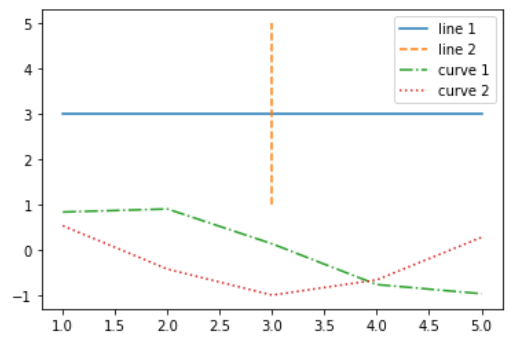Whether you're preparing for your first job interview or aiming to upskill in this ever-evolving tech landscape, GeeksforGeeks Courses are your key to success. We provide top-quality content at affordable prices, all geared towards accelerating your growth in a time-bound manner. Join the millions we've already empowered, and we're here to do the same for you. Don't miss out - check it out now!

Previous
Next